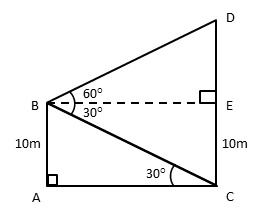# CBSE Class 10 Math Question Paper 2016 | Q19

###### Applications of Trigonometry | 3 Mark | Heights & DistancesClass 10 Maths Questions

The given question is a medium difficulty 3-mark question from the chapter Applications of Trigonometry. Applying trigometric ratios to compute two sides of right triangles. It is an important question and appeared in Section C of the 2016 CBSE class 10 board paper.

Question 19: A man standing on the deck of a ship, which is 10 m above water level, observes the angle of elevation of the top of a hill as 60° and the angle of depression of the base of hill as 30°. Find the distance of the hill from the ship and the height of the hill.

## NCERT Solution to Class 10 Maths

### Explanatory Answer | CBSE 2016 Maths Paper Q19Let AB be the deck of the ship that is 10m above water level.
Let AC be the distance between the hill and the deck of the ship.
Let CD be the hill.

Draw BE parallel to AC.
∠EBD is the angle of elevation of the top of the hill from the deck of the ship = 60°
∠EBC is the angle of depression of the base of the hill from the deck of the ship = 30°
Because BE and AC are parallel, ∠EBC = ∠BCA = 30°

##### Step 1: Compute AC, distance between ship and the hill.

In ΔBAC, tan C = $\frac{\text{opposite side}}{\text{adjacent side}}$ = $\frac{AB}{AC}$
AB = CE = 10 m
tan 30° = $\frac{10}{AC}$
tan 30° = $\frac{1}{\sqrt3}$
∴ $\frac{1}{\sqrt3}$ = $\frac{10}{AC}$ or AC = 10$\sqrt{3}$
AC is the distance between the ship and the hill = 10$\sqrt{3}$ m

##### Step 2: Compute DE and then height of hill.

In Δ BED, tan B = $\frac{\text{opposite side}}{\text{adjacent side}}$ = $\frac{DE}{BE}$
But BE = AC = 10$\sqrt{3}$ m
tan 60° = $\frac{DE}{AC}$
tan 60° = $\sqrt{3}$
So, $\sqrt{3}$ = $\frac{DE}{10\sqrt{3}}$
∴ DE = 10$\sqrt{3}$ × $\sqrt{3}$ = 30 m

Height of the hill = DC = DE + EC
= 30 + 10 = 40 m

###### Try CBSE Online CoachingClass 10 Maths

Register in 2 easy steps and
Start learning in 5 minutes!